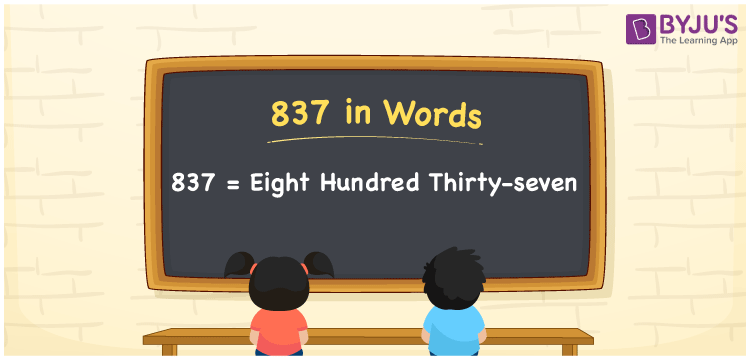# 837 in Words

We can write 837 in words as Eight hundred thirty-seven. If you purchased a sports plaything at Rs. 837, then you can say, “I purchased a sports plaything for Eight hundred thirty-seven rupees”. From this statement, we can say that Eight hundred thirty-seven is the number name or word form of 837. Also, note that 837 is a cardinal number since it conveys a specific amount.

 837 in words Eight hundred thirty-seven Eight hundred thirty-seven in Numbers 837

## 837 in English Words

We generally write numbers in words using the English alphabet. So, we spell 837 in English words as “Eight hundred thirty-seven”.## How to Write 837 in Words?

Learn how to write the number 837 in words using a place value chart with three columns, given below.

 Hundreds Tens Ones 8 3 7

Here, ones = 7, tens = 3, hundreds = 8

So, 8 × Hundred + 3 × Ten + 7 × One

= 8 × 100 + 3 × 10 + 7 × 1

= 800 + 30 + 7

= Eight hundred + Thirty + Seven

= Eight hundred thirty-seven

Therefore, 837 in words is written as Eight hundred thirty-seven.

837 is a natural number that precedes 838 and succeeds 836.

837 in words – Eight hundred thirty-seven

Is 837 an even number? – No

Is 837 an odd number? – Yes

Is 837 a prime number? – No

Is 837 a composite number? – Yes

Is 837 a perfect square number? – No

Is 837 a perfect cube number? – No

## Frequently Asked Questions on 837 in Words

Q1

### How do you spell 837?

You can spell the number 837 in English words as Eight hundred thirty-seven.
Q2

### Write Rs. 837 in words.

An amount of Rs. 837 can be written in words as Eight hundred thirty-seven rupees.
Q3

### What is the value of 1 + 837 in words?

1 + 837 = 838 Threfore, the value of 1 + 837, i.e., 838 in words is Eight hundred thirty-eight.